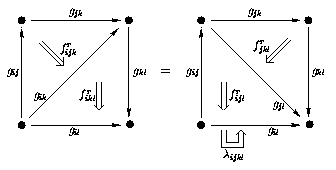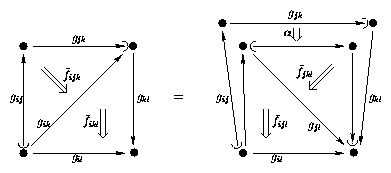## April 15, 2005

### Weak NABG

#### Posted by ursOn USENET David Roberts has mentioned some issues related with the desire to have weakened nonabelian bundle gerbes. It is not immediately clear what this would be, but the 2-bundle perspective might give a hint.The fact that principal strict $\mathrm{Aut}\left(G\right)$-2-bundles over base 1-spaces encode the same information as a nonabelian (bundle) $G$-gerbes (NABG) suggests that there should be a weakened version of them, since there is a natural notion of weak 2-bundle and no good reason to restrict attention to strict ones.

In a strict 2-bundle the ordinary transition law

(1)${g}_{\mathrm{ij}}{g}_{\mathrm{jk}}={g}_{\mathrm{ik}}$

is replaced by the existence of a 2-group isomorphism

(2)${g}_{\mathrm{ij}}{g}_{\mathrm{jk}}\stackrel{{\overline{f}}_{\mathrm{ijk}}}{\to }{g}_{\mathrm{ik}}$

which has to satisfy the following coherence law:This ensures that using ${f}_{\dots }$ to simplify multiple products of ${g}_{\dots }$ is independent of the precise way one does this. So in a sense this ensures that we weaken only one level, not more.

Using the rules of strict 2-groups and in particular the fact that a strict 2-group is nothing but a crossed module the above diagram is immediately seen to be equivalent to the usual cocycle condition known for nonabelian gerbes:

(3)${f}_{\mathrm{ijk}}{f}_{\mathrm{ikl}}=\alpha \left({g}_{\mathrm{ij}}\right)\left({f}_{\mathrm{jkl}}\right){f}_{\mathrm{ijl}}\phantom{\rule{thinmathspace}{0ex}}.$

(Here and in the following I assume for convenience that the twist vanishes so that $\lambda =1$.)

The generalization of the above situation to weak 2-groups is straightforward at the level of diagrams. For a weak 2-group associativity

(4)$\left({g}_{\mathrm{ij}}{g}_{\mathrm{jk}}\right){g}_{\mathrm{kl}}={g}_{\mathrm{ij}}\left({g}_{\mathrm{jk}}{g}_{\mathrm{kl}}\right)$

is replaced by an isomorphism

(5)$\left({g}_{\mathrm{ij}}{g}_{\mathrm{jk}}\right){g}_{\mathrm{kl}}\stackrel{{\alpha }_{\mathrm{ijkl}}}{\to }{g}_{\mathrm{ij}}\left({g}_{\mathrm{jk}}{g}_{\mathrm{kl}}\right)$

called the associator.

This has to be inserted in the above diagram to rebracket the product of the ${g}_{\dots }$. The more general diagram then looks like this:(Note here that semi-circles on the edges indicate the order in which the product is performed.)

So this is the diagrammatic version of the cocycle condition describing a weak 2-bundle (over a base 1-space), and hence something like this is to be expected to be necessary for weak nonabelian bundle gerbes, too.

It is easy enough to write this down. But since there is no known analog of the relation

strict 2-group $↔$ crossed module

for weak 2-groups, it is hard to say what the above would mean algebraically.

One can work out what the corresponding ‘weak crossed modules’ would be like, but it seems to be a mess of properties that look much more complicated than the above diagram.

Posted at April 15, 2005 3:56 PM UTC

TrackBack URL for this Entry:   http://golem.ph.utexas.edu/cgi-bin/MT-3.0/dxy-tb.fcgi/555

### Re: Weak NABG

On a related note, does anyone know where the T_A(h) term in the bitorsor connection pullback equation

l^h* a = Ad_h a + p*T_A(h)

in Aschieri-Cantini-Jurco (hep-th/0312154) comes from? Here l^h is left multiplication by h in our group G, a is the Lie(G)-valued 1-form and A is the Lie(Aut(G))-valued 1-form.

I know it’s related to the a_ij in

A_j + a_ij = gA_ig^{-1} + g^{-1}dg

(with the obvious indices on the g’s), but it seems to be pulled out of the air in the above paper. I know we need it to retain left-right symmetry in the bitorsor but the direction ‘see later’ halfway down page 11 isn’t helpful.

David

Posted by: David Roberts on April 20, 2005 7:30 AM | Permalink | Reply to this

### Re: Weak NABG

I believe the argument is just that this term can be included, since it does not break the left-right symmetry. I am not aware of a more systematic derivation, except perhaps that this way the same cocycles are reproduced which Breen & Messing obtain, too.

I might be wrong, though. I’ll check with Branislav Jurčo.

(I note that without this term we get a field strength valued in the center of $\mathrm{Lie}\left(H\right)$, as we do if we want to have a strict 2-holonomy 2-functor. But I haven’t figured out yet if that’s more than a coincidence.)

Posted by: Urs Schreiber on April 20, 2005 11:21 AM | Permalink | Reply to this

### Exponentiating weak Lie 2-algebrasI have thought a little more about the general problem addressed in the above entry. Maybe it is possible to proceed this way:

While it is hard to deal with weak Lie 2-groups, it is easy to deal with weak (i.e. semistrict) Lie 2-algebras. These are nothing but 2-term ${L}_{\infty }$ algebras in a different guise and have a simple list of properties. (See for instance lemma 33 of HDA6 or section 2.2 in our ‘From Loop Groups to 2-Groups’).

So here a Lie 2-algebra element (a morphism in the 2-algebra) is a couple

(1)$\left(x,\stackrel{⇀}{f}\right)$

with $x\in {V}_{0}$ and $\stackrel{⇀}{f}\in {V}_{1}$ elements of two vector spaces, and the bracket on such couples is

(2)$\left[\left({x}_{1},{\stackrel{⇀}{f}}_{1}\right),\left({x}_{2},{\stackrel{⇀}{f}}_{2}\right),\right]=\left({l}_{2}\left({x}_{1},{x}_{2}\right),\phantom{\rule{thinmathspace}{0ex}}{l}_{2}\left({x}_{1},{\stackrel{⇀}{f}}_{2}\right)-{l}_{2}\left({x}_{2},{\stackrel{⇀}{f}}_{1}\right)+{l}_{2}\left(d{\stackrel{⇀}{f}}_{1},{\stackrel{⇀}{f}}_{2}\right)\right)$

where

(3)${l}_{2}\left({V}_{0},{V}_{0}\right)={V}_{0}$

and

(4)${l}_{2}\left({V}_{0},{V}_{1}\right)={V}_{1}$

is the binary operation in the ${L}_{\infty }$ algebra and

(5)${V}_{1}\stackrel{d}{\to }{V}_{0}$

is our 2-term ‘complex’.

Given any such (2-)algebra $ℒ$ with a bracket we can try to ‘exponentiate’ it to obtain an algebra we might call $\mathrm{exp}\left(ℒ\right)$ as follows:

Let the the elements of $\mathrm{exp}\left(ℒ\right)$ be formal symbols

(6)$\mathrm{exp}\left(x,\stackrel{⇀}{f}\right)$

for each $\left(x,\stackrel{⇀}{f}\right)\in ℒ$ and define the algebra product by means of the Baker-Campbell-Hausdorff formula as

(7)$\mathrm{exp}\left({x}_{1},{\stackrel{⇀}{f}}_{1}\right)\mathrm{exp}\left({x}_{2},{\stackrel{⇀}{f}}_{2}\right)=\mathrm{exp}\left(\left({x}_{1},{\stackrel{⇀}{f}}_{1}\right)+\left({x}_{2},{\stackrel{⇀}{f}}_{2}\right)+\frac{1}{2}\left[\left({x}_{1},{\stackrel{⇀}{f}}_{1}\right),\left({x}_{2},{\stackrel{⇀}{f}}_{2}\right)\right]+\frac{1}{12}\left[\left({x}_{1},{\stackrel{⇀}{f}}_{1}\right)-\left({x}_{2},{\stackrel{⇀}{f}}_{2}\right),\phantom{\rule{thinmathspace}{0ex}}\left[\left({x}_{1},{\stackrel{⇀}{f}}_{1}\right),\left({x}_{2},{\stackrel{⇀}{f}}_{2}\right)\right]\right]+\cdots \right)\phantom{\rule{thinmathspace}{0ex}}.$

when $ℒ$ is the 2-term ${L}_{\infty }$ algebra of a strict Lie 2-algebra (which then is nothing but a differential crossed module) then $\mathrm{exp}\left(ℒ\right)$ is nothing but the algebra under the horizontal product of a strict 2-group (i.e. a crossed module).

More generally we have a non-vanishing Jacobiator

(8)${l}_{2}\left(x,{l}_{2}\left(y,z\right)\right)+{l}_{2}\left(z,{l}_{2}\left(x,y\right)\right)+{l}_{2}\left(y,{l}_{2}\left(z,x\right)\right)={\mathrm{dl}}_{3}\left(x,y,z\right)$

(for $x,y,z\in {V}_{0}$) and find that the product in $\mathrm{exp}\left(ℒ\right)$ is non-associative:

On the one hand we have

(9)$\left({e}^{\left(x,0\right)}{e}^{\left(y,0\right)}\right){e}^{\left(z,0\right)}=\mathrm{exp}\left(x+y+z-\frac{1}{12}{\mathrm{dl}}_{3}\left(x,y,z\right)+\cdots \phantom{\rule{thinmathspace}{0ex}},\phantom{\rule{thinmathspace}{0ex}}0\right)$

on the other

(10)${e}^{\left(x,0\right)}\left({e}^{\left(y,0\right)}{e}^{\left(z,0\right)}\right)=\mathrm{exp}\left(x+y+z+\frac{1}{12}{\mathrm{dl}}_{3}\left(x,y,z\right)+\cdots \phantom{\rule{thinmathspace}{0ex}},\phantom{\rule{thinmathspace}{0ex}}0\right)\phantom{\rule{thinmathspace}{0ex}}.$

Hence there would be an associator

(11)$\left({e}^{x}\otimes {e}^{y}\right)\otimes {e}^{z}\stackrel{{\alpha }_{{e}^{x},{e}^{y},{e}^{z}}}{\to }{e}^{x}\otimes \left({e}^{y}\otimes {e}^{z}\right)$

given to lowest order by

(12)${\alpha }_{{e}^{x},{e}^{y},{e}^{z}}\approx \left(\left({e}^{x}{e}^{y}\right){e}^{z}\right)\mathrm{exp}\left(0,\frac{1}{6}{l}_{3}\left(x,y,z\right)\right)$

if we (as necessary to get the associative case right) declare the target of $\mathrm{exp}\left(0,\stackrel{⇀}{f}\right)$ to be

(13)$t\left(\mathrm{exp}\left(0,\stackrel{⇀}{f}\right)\right)={e}^{d\stackrel{⇀}{f}}\phantom{\rule{thinmathspace}{0ex}}.$

I haven’t checked if and how this associator extends to all orders.

One more condition I have checked though is the following: Given the above horizontal product, the exchange law in the weak 2-group to-be uniquely specifies the vertical product of $\mathrm{exp}\left({x}_{1},{\stackrel{⇀}{f}}_{1}\right)$ with $\mathrm{exp}\left({x}_{2},{\stackrel{⇀}{f}}_{2}\right)$. This, however, has to be associative, since it must be just composition of arrows in a category. By working it out one finds that this implies that

(14)${l}_{3}\left(d\stackrel{⇀}{f},d\stackrel{⇀}{g},\stackrel{⇀}{h}\right)=0$

for all $\stackrel{⇀}{f},\stackrel{⇀}{g},\stackrel{⇀}{h}\in {V}_{1}$.

I am not sure how drastic this restriction cuts down the space of all interesting ${L}_{\infty }$ algebras, though.

Anyway, if we restrict attention to ${L}_{\infty }$-algebras that do satisfy this the above might be a way to get a handle on weak 2-groups and hence possibly on weakened nonabelian bundle gerbes.

P.S.

For more information on how to use the MathML support of this weblog see this.

Posted by: Urs Schreiber on April 20, 2005 12:18 PM | Permalink | Reply to this

Post a New Comment